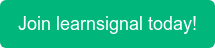##### What is Put Call Parity?

Put-call parity is considered a critical aspect of option pricing. It allows investors and risk managers to calculate the price of either put or call if the value of anyone is already provided. The put-call parity is derived based on two options strategies: a fiduciary call and a protective put.

A fiduciary call combines a zero-coupon bond that pays X at maturity and a call option with an exercise price of X. The payoff for a fiduciary call at expiration is X when the call is out-of-the-money, and X + (S − X) = S when the call is in the money.

Now, let's discuss the protective put, a combination of stock and a put option on the stock. The expiration date payoff for a protective put is (X − S) + S = X when the put is in-the-money, and S when the put is out-of-the-money.

##### Example of Put Call Parity:

Put call parity is defined at

p = c − S + PV(X)

Let's assume a combination of options with a call price of USD 10, a stock price of USD 50 and the present value of a bond is given as USD 90. Given this, we can calculate the price of the put option as below:

P = 10 – 50 + 90 = 50

Topics: ACCA, CIMA, CPD, AAT, FRM•### Central Counterparties

•### Country Risk

•### Convexity Formula

•### Clean and Dirty Price

•### Basic Indicator Approach

•### ACCA Exam Fees 2022: Everything You Need to Know

•### ACCA Exam Dates for 2022

•### How Difficult is Passing ACCA? An Honest Review

•### 7 of the Best YouTube Channels for Accounting Students

•